## Monday, March 19, 2007

### Final report

My final report is available here (follow the link). I definitely enjoyed to work hard on this project. I also learned a lot from the others CSE190a projects. Thank you all!

## Wednesday, March 14, 2007

### Final review

Here is a link to the final presentation. It summarizes the overall approach of the project and the results I got.

## Wednesday, March 7, 2007

### Feature correspondence

As NCC and SIFT are not suited to find correspondences in fingerprints (a minutia point looks like an other minutia point), I tried to design my own algorithm. Serge advised me to imitate what humans do when tring to find correspondences between two sets of points: here is the simple algorithm I wrote.

The overall idea is to compute for each point in the two sets, a feature vector, and then compare the features using a feature distance.

Let's consider a minutia point M. The main idea, shown on the next picture, is to consider the 3 closest points around M, we can call them M1 M2 and M3. The distance between M and M' will be found using the euclidean distance between M1,M2,M3 and M'1,M'2,M'3. This can not be done directly and a few adjustments need to be done.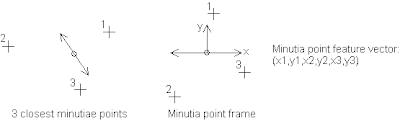First of all, the orientation of the points may not be the same due to the print rotation. We need first to rotate all the points of an angle equal to the ridge orientation of M.
The indexing of the points may not correspond: for example M1<->M'3, M2<->M'1, M3<->M'2. So we need to compute the sum of the distances between the 3 correspondences, for all the permutations of the points: we then take the minimum of these. If the minimum is small, it means that the 3 points around M are in the same relative position as the 3 points around M'.

This is an algorithm based on 3 points assuming that there is no false minutia point. A 1 point distance, that can allow 2 false minutiae points is simply the minimum over all the distances between the points. If for example only M2<->M'3, then the distance will be small.

I used an indermediate 2 points distance, which gives quite good results. This is based on the same idea but for 2 points: it is the minimum of the sum of the distances between every two pairs of correspondences. For example: M1<->M'3, M2<->M'1 (M3 and M'2 may be far away from eachother). This method combines the advantages from the 1 and 3 points distance (robust to noise and accurate).

For the final step, I compared for each minutia point M the distance to the minutiae points in the other set and took the 5 smallest distances: those 5 points are said to be the the correspondences of M. This is then fed to Ransac, which greatly help to find quickly a solution (a solution is found, when there is one, in about 100 iterations).

This week end I also implemented the refinement method for Ransac. It helped sometimes to go over the limit of 10 matching points in both cases (false accept and true reject).

The next step is to tune a bit more the algorithm and run it on the testing set. I will be able to do 100x100 comparisons, but not 600x600.

As an hall of fame: I found 2 prints in my training set that are said to come from the same finger, but cannot see any similarity.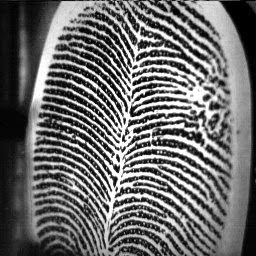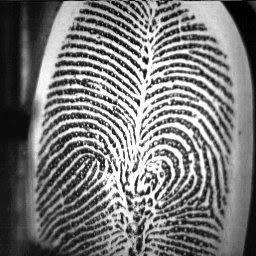## Saturday, February 24, 2007

### Minutiae points detection and matching

I have been working on minutiae points detection using template matching. The goal was to find a filter that, when applied on the enhanced print, pops out the bifurcation and ridge endings.

After trying some real minutia point template in various direction, I decided to create a template using maths functions. The main motivation is because it allows to orient the template in any direction without problems in filling the holes of the rotation. It is also convenient because it can be tuned easily. I did not remember having seen this kind of approach in the literature or the net, but it is attractive and quite powerful. I used interpolated cosine wave functions with different frequencies.

Due to the duality of ridge ending and bifurcation, the enhanced image has to be filtered using two filters. This can be done by inverting the image, or the filter, or rotating the filter of 180°. I chose the first solution even if it is not the faster one. Then the local maxima of the two filtered images need to be extracted and merged together (a threshold can be adjusted here). The next picture shows all those steps.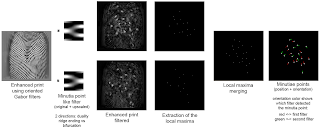After having the minutiae point position and direction, I tryed Ransac on different prints even if I do not specify correspondences. The next picture shows that the two fingerprints are considered to match.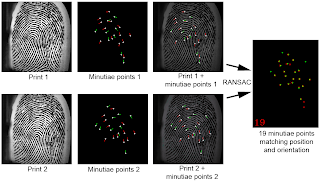I also made functions to save and load minutiae points from a text file, which allows me to begin some tests. The first impression is that is is very slow, because I compute for each pixel the corresponding oriented Gabor filter and minutia template. It takes 15 seconds to enroll a print. It also take 10 seconds to do 5000 iterations of Ransac. An other thing that can be improved is to use pointers and increment them instead of using the convenient function cvGetReal2D(img, i, j). The second impression is that I do not have false accepts, but some true rejects. That is because I mainly tune the program on good quality prints. Also Ransac need correspondences to work accurately and decrease those rates.

Next steps:
- Find correspondences: I tryed to run David Lowe's SIFT algorithm, but it gives features of 1 pixel size. Moreover, It founds more than 2000 features. I may also try Normalized Cross Correlation.
- Do the draft report.
- Test and tune the program.

## Monday, February 12, 2007

### Gabor filters are cool

A picture worth more than a thousand words, so I am going to explain briefly what I did, and then show the results.

- I tryed SVD on the covariance matrix of the gradient versus averaging the gradient, and SVD gives better results. It provides an estimation of the reliabilit
y of the orientation (using the ratio of the two eigenvalues). The ridge orientation is given by the orientation of the vector having the smallest eigenvalue (which is positive because the covariance matrix is symetric).

- I change the color legend used to plot the orientation. HSV color space is used: Hue for the orientation (0°=red, 120°=green and so on), Saturation is maximum (full color), and Value is the max eigenvalue normalised in the overall image (I notice writting this post that it needs to be the ratio of the eigenvalues instead). Orientation directions are the same length on the picture.

- In order to get the orientation for each pixel in the image, orientation is bilinearly interplolated using the orientation computed on the small windows.

- Finally, I applied Gabor filters to enhance the fingerprint. For each pixel, given the orientation, I compute the Gabor filter having that direction (it is really expensive, but I was lazy to precompute a bank of filters). This gives a pretty neat nearly black and white image, where ridges are clearly visible. As the authors of "the handbook of fingerprint recognition" advised, I fixed the standard deviation of the gaussian in x and y to 4. I used a sine wave instead of a cosine (against what is used in the book), so that the average value of the filter is 0. The frequency of the sine wave can be set, but I did not estimate the local ridge frequency. I will do that later on.

Here is the picture summing up the results: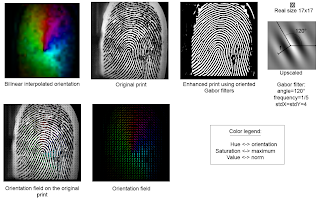## Wednesday, February 7, 2007

### Ridge Orientation

First of all, since minutiae points detection has to be trainned using fingerprints, I separate evenly my database into the trainning set and the testing set (about 600 prints each). I then separate the trainning set into 2 subsets, which will allow me to do trainning-testing cycles without using the original testing set. The fingerprints were downloaded from the FVC (Fingerprint Verification Competition). Of course, I will not use the testing set until the end of the project.

Then, the first set in minutia detection is to estimate rigde field orientation. This allows to find the local orientation of the ridges. In the handbook of fingerprint recognition, several methods are presented. I programed the first one, which is directly inspired from CSE166.

Using Sobel filter, I compute the gradient in x and y of the image (Ix and Iy). Then, for each pixel in the image, I can compute the orientation and the norm of the gradient and plot it. The orientation is a number between 0° and 180° representing the angle from the x axis. Since it is influenced by noise and difficult to plot, we need to compute an average inside a small window (let's say 8x8). A trick is used to average the angles: because we don't want that the average of 10° and 170° be 90°, the angles are doubled. Averaging 20° and 340° gives 180°, which is the right direction angle (same direction as 0°).

One thing to notice is that the orientation given by the formula atan(Iy/Ix), is not the direction of the ridge. We need to add Pi/2 to it.

I had some problems to plot the direction of the minutiae points. I was first testing it on a circle image: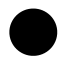After I got it working right, I then tryed it on a fingerprint using a 4x4 window. Here are the original print, the direction field thresholded, and height map (the norm field).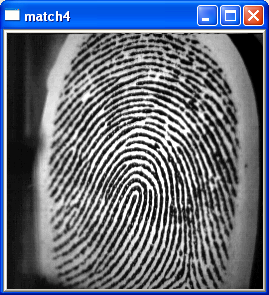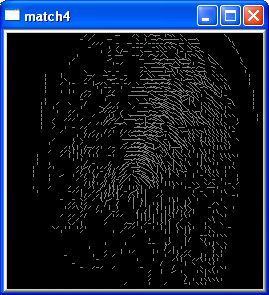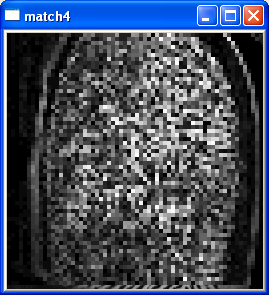Some issues to test:

- what is the right size of the small window?
- Using an other method, based on SVD on the covariance matrix of the gradient.

## Monday, January 29, 2007

### Ransac in action

Here is a video showing how ransac works. After some iterations, the right transformation is found (does not show up well at the end of the video).

Sometimes, after the transformation, all the points are aligned, or shrinked onto one point. Thus the number of matches may be high. To avoid that problem, I verify that the transformation spread the points by computing the determinant of A (the rotation, scaling, shearing matrix). If is under 0.1, It means that the transformation is not valid, and it doesn't worth counting the number of matches.

I choose to compute only the determinant and not the ratio between the norm of eigen values because it is quite expensive. This may be added later if needed. From now, all my test sets involve rigid transformation (rotation+translation), so the determinant is equal (or close) to 1. I think there will be some scaling effect between the natural fingerprints I have, but It may not be too important (the ratio may stay between 0.5 and 2).

Then, I generated an other print in two position. For each position, I made 5 groups of minutiae points (colored in red, blue, cyan, green and yellow), that are the corresponding points.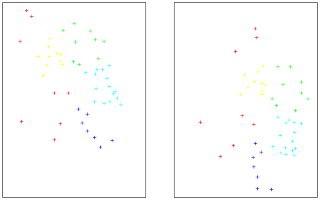The colors allow to load an image and run ransac on it. It does a good job by finding the transformation (not shown here).
I then delete about 2-3 points in every set and every group of colors: in each picture, 13 points are thrown away. Initially, there were 49 points in each set, now there are only 49-2x13=23 corresponding points and 26 outliers (13 in each set). Ransac do not care about outliers and still finds the transformation as this picture shows.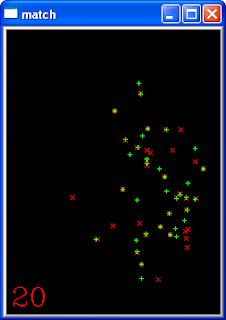I will explain later the statistics involved in ransac algorithm, why it is important to find a small subset of correspondences, how estimate the error we can make (false acceptance and false rejection).

I still need to refine the transformation using matching points (see if it increases the number of points), and then I am going to begin the detection of minutiae points and creation of a feature vector. I will need to have a closer look to the fingerprint database, and separate evenly in two subsets: one for the testing, one for the training.